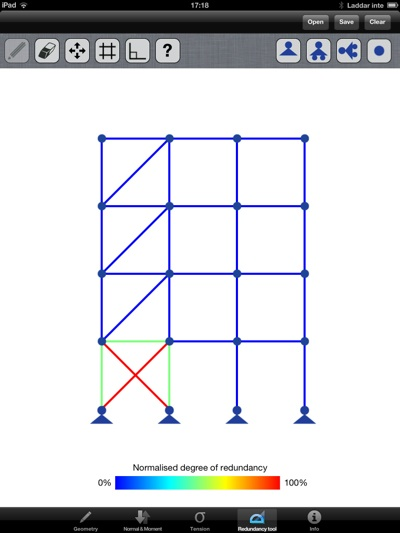# Sketch a Frame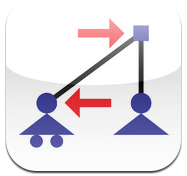## Introduction

Sketch a Frame is a finite element software tool for the iPad. The tool is developed for the early stage design process and for educational puposes. Gestures are used for modelling, and calculations are perfomed as the model is stable. The software has four different modes; geometry, normal force and moment, tension and the redundancy tool. If the model is not stable the modal shape is visualised which helps the user to understand how to stabilise the model.

This is a master thesis work by Daniel Åkesson at Lunds University, read the full thesis.### Geometry mode

When the model is stable the deformation shape is visualised. This is automatically scaled as changes are applied.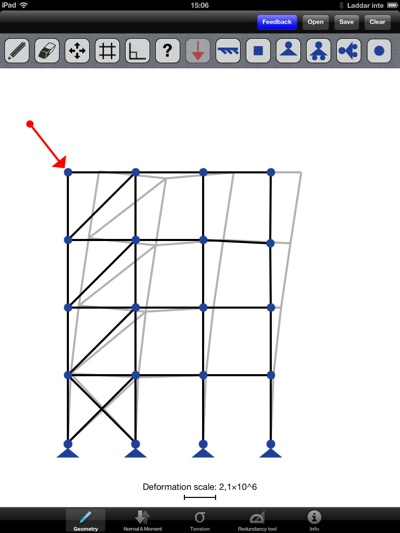### Normal force & moment mode

The normal force is a measure of the force in the direction of the beam. Red represents tension and blue compression.

If the model has a moment this is visualised in this mode.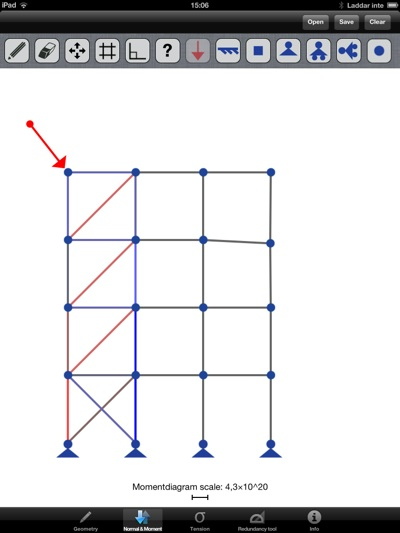### Tension mode

The tension is calculated by N/A+M/W using material parameters from an IPE-80 beam. The tension is normalised and visualised from black 0 % to red 100 % of relative tension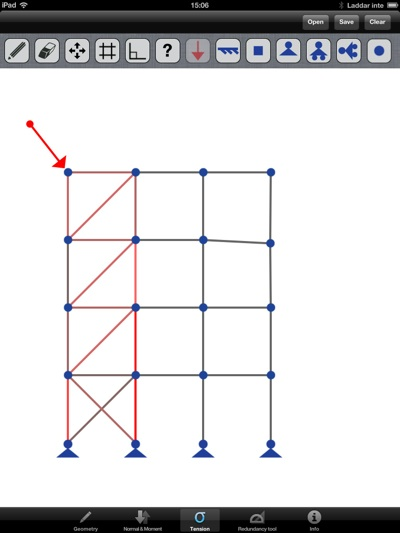### Redundancy mode

A normalised redudancy of 0 % (blue) means that the element is cannot be removed as its removal would render the framwork unstable. A factor of 100 % (red) means that the element is highly redundant. The redundancy analysis is made without considering a specific load case thus only topology, geometry and constraints are considered. Based on the work of Gunnar Tibert, article found here under "Redundancy factors in design".